correlation analysis

• ## 相关性分析

千次阅读 2018-08-28 10:19:43
而在探索性分析的各种招式中，相关性分析往往有着一招致敌的奇效，下面简单地介绍一些常见的相关系数。 相关性分析 顾名思义，相关性分析旨在研究两个或两个以上随机变量之间相互依存关系的方向和密切程度，从多...
目录

相关性分析
从随机变量之间的关系角度
从随机变量自身的分布类型角度

相关性检验
Pearson(皮尔逊相关系数)
Spearman(秩相关系数)
Kendall Rank(肯德尔相关系数)
Kappa一致性系数
卡方检验
Fisher精确检验
Cochran-Mantel-Haenszel（简称CMH检验）

“工欲善其事，必先利其器”，如果把数据建模比作伐木，那么前期的数据探索性分析则是细致的“霍霍磨刀”，有效的探索性分析能够帮助我们捕捉到数据之间的关联效应，为之后的特征工程、模型构建奠定基础。而在探索性分析的各种招式中，相关性分析往往有着一招致敌的奇效，下面简单地介绍一些常见的相关系数。

相关性分析

顾名思义，相关性分析旨在研究两个或两个以上随机变量之间相互依存关系的方向和密切程度，从多个角度立体地剖析相关性分析有助于我们更立体地掌握相关性分析：

从随机变量之间的关系角度

线性相关(Pearson Spearman Rank Kendall Rank)
非线性相关

从随机变量自身的分布类型角度

连续变量与连续变量(Pearson Spearman Rank Kendall Rank)
连续变量与分类变量(Spearman Rank Kendall Rank)

相关性检验

Pearson(皮尔逊相关系数)

皮尔逊相关系数适用于：

两个变量之间是线性关系，都是连续数据；
两个变量的总体是正态分布，或接近正态的单峰分布；
两个变量的观测值是成对的，每对观测值之间相互独立。

随机变量、的皮尔逊相关系数计算公式如下：

ρX,Y=Cov(X,Y)σXσY${\rho }_{X,Y}=\frac{Cov\left(X,Y\right)}{{\sigma }_{X}{\sigma }_{Y}}$\rho_{X,Y}=\frac{Cov(X,Y)}{\sigma_X\sigma_Y}

Cov(X,Y)$Cov\left(X,Y\right)$$Cov(X,Y)$为随机变量X$X$$X$、Y$Y$$Y$的协方差，(σX,σY)$\left({\sigma }_{X},{\sigma }_{Y}\right)$$(\sigma_X,\sigma_Y)$分别表示随机变量X$X$$X$、Y$Y$$Y$的标准差，ρX,Y∈[0,1]${\rho }_{X,Y}\in \left[0,1\right]$$\rho_{X,Y}\in[0,1]$，越大代表随机相关性越强。

Spearman(秩相关系数)

Spearman秩相关系数适用于：

随机变量是成对的有序分类变量；
不论数据分布，数据呈单调关系，可以度量随机变量的非线性关系。

随机变量X$X$$X$、Y$Y$$Y$的Spearman秩相关系数ρS${\rho }_{S}$$\rho_{S}$计算如下，对原始随机变量xi${x}_{i}$$x_i$ 、yi${y}_{i}$$y_i$降序排序，记dxi${d}_{i}^{x}$$d_i^x$、dyi${d}_{i}^{y}$$d_i^y$为原始xi${x}_{i}$$x_i$ 、yi${y}_{i}$$y_i$在排序后列表中的位置，dxi${d}_{i}^{x}$$d_i^x$、dyi${d}_{i}^{y}$$d_i^y$称为xi${x}_{i}$$x_i$ 、yi${y}_{i}$$y_i$的秩次，若有一个变量或两个变量的值对应相等，则该对观测值是相持的，相持还分为在X$X$$X$（极为Tx${T}_{x}$$T_x$）或相持在变量Y$Y$$Y$上（记为Ty${T}_{y}$$T_y$），秩次差di=dxi−dyi${d}_{i}={d}_{i}^{x}-{d}_{i}^{y}$$d_i=d_i^x-d_i^y$。Spearman秩相关系数为:

没有相持等级
ρS=1−6∑d2in(n2−1)${\rho }_{S}=1-\frac{6\sum {d}_{i}^{2}}{n\left({n}^{2}-1\right)}$\rho_S=1-\frac{6\sum d_i^2 }{n(n^2-1)}
有相持等级
ρS=∑x2+∑y2−∑d2i2∑x2∑y2‾‾‾‾‾‾‾‾‾‾√,∑x2=N3−N12−∑Tx,∑y2=N3−N12−∑Ty${\rho }_{S}=\frac{\sum {x}^{2}+\sum {y}^{2}-\sum {d}_{i}^{2}}{2\sqrt{\sum {x}^{2}\sum {y}^{2}}},\sum {x}^{2}=\frac{{N}^{3}-N}{12}-\sum {T}_{x},\sum {y}^{2}=\frac{{N}^{3}-N}{12}-\sum {T}_{y}$\rho_S=\frac{\sum x^2 +\sum y^2 -\sum d_i^2}{2\sqrt{\sum x^2\sum y^2}},\sum x^2=\frac{N^3-N}{12}-\sum T_x,\sum y^2=\frac{N^3-N}{12}-\sum T_y

举个例子：

xi${x}_{i}$$x_i$
yi${y}_{i}$$y_i$
dxi${d}_{i}^{x}$$d_i^x$
dyi${d}_{i}^{y}$$d_i^y$
di${d}_{i}$$d_i$

4
7
5
6
1

44
67
1
1
0

15
9
4
5
1

23
54
2
2
0

18
21
3
4
1

2
33
6
3
-3

对于上表数据，算出Spearman秩相关系数为：1-6*(1+1+1+9)/(6*35)=0.6571

Kendall Rank(肯德尔相关系数)

肯德尔相关系数适用于：

随机变量是成对的有序分类变量
不论数据分布，数据呈单调关系，可以度量随机变量的非线性关系。

肯德尔系数的定义：n$n$$n$个同类的统计对象按特定属性排序，其他属性通常是乱序的。P$P$$P$为和谐对（变量大小顺序相同的样本观测值）的个数、Q$Q$$Q$为f$f$$f$不和谐对（变量大小顺序相同的样本观测值），n$n$$n$为随机变量容量。一对观测值中，若有一个变量或两个变量的值对应相等，则该对观测值是相持的，相持还分为在X$X$$X$（记为Tx${T}_{x}$$T_x$）或相持在变量上Y$Y$$Y$（记为Ty${T}_{y}$$T_y$ ），因此公式可以表示为：

没有相持等级

τb=2(P−Q)n(n−1)${\tau }_{b}=\frac{2\left(P-Q\right)}{n\left(n-1\right)}$\tau_b=\frac{2(P-Q)}{n(n-1)}

有相持等级

τb=P−Q(P+Q+Tx)(P+Q+Ty)‾‾‾‾‾‾‾‾‾‾‾‾‾‾‾‾‾‾‾‾‾‾‾‾√${\tau }_{b}=\frac{P-Q}{\sqrt{\left(P+Q+{T}_{x}\right)\left(P+Q+{T}_{y}\right)}}$\tau_b=\frac{P-Q}{\sqrt{(P+Q+T_x)(P+Q+T_y)}}

Kappa一致性系数

Kappa一致性系数适用于：

随机变量是成对的分类变量

随机变量X$X$$X$、Y$Y$$Y$的Kappa$Kappa$$Kappa$一致性系数K$K$$K$计算公式如下：

K=P(A)−P(E)1−P(E),P(A)=1NK(K−1)∑i=1N∑j=1mn2ij−1K−1,P(E)=∑j=1mP2j,Pj=CjNK,Cj=∑i=1Nnij$K=\frac{P\left(A\right)-P\left(E\right)}{1-P\left(E\right)},P\left(A\right)=\frac{1}{NK\left(K-1\right)}\sum _{i=1}^{N}\sum _{j=1}^{m}{n}_{ij}^{2}-\frac{1}{K-1},P\left(E\right)=\sum _{j=1}^{m}{P}_{j}^{2},{P}_{j}=\frac{{C}_{j}}{NK},{C}_{j}=\sum _{i=1}^{N}{n}_{ij}$K=\frac{P(A)-P(E)}{1-P(E)},P(A)=\frac{1}{NK(K-1)}\sum^N_{i=1}\sum^m_{j=1}n_{ij}^2-\frac{1}{K-1},P(E)=\sum^m_{j=1}P_j^2,P_j=\frac{C_j}{NK},C_j=\sum^N_{i=1}n_{ij}

N$N$$N$为随机变量容量，K$K$$K$为一致预测，nij${n}_{ij}$$n_{ij}$为细格预测数，也可以表示为：
K=p0−pc1−pc$K=\frac{{p}_{0}-{p}_{c}}{1-{p}_{c}}$K=\frac{p_0-p_c}{1-p_c}

举例：

预测\实际
A$A$$A$
B$B$$B$
C$C$$C$

A$A$$A$
239
21
16

B$B$$B$
16
73
4

C$C$$C$
6
9
280

p0=239+73+280664=0.8916,pc=261∗276+103∗93+300∗295664∗664=0.3883,K=0.8916−0.38831−0.3883${p}_{0}=\frac{239+73+280}{664}=0.8916,{p}_{c}=\frac{261\ast 276+103\ast 93+300\ast 295}{664\ast 664}=0.3883,K=\frac{0.8916-0.3883}{1-0.3883}$$p_0=\frac{239+73+280}{664}=0.8916,p_c=\frac{261*276+103*93+300*295}{664*664}=0.3883,K=\frac{0.8916-0.3883}{1-0.3883}$

卡方检验

卡方检验适用于：

随机变量是成对的分类变量；
最好是大样本数据。一般每个个案最好出现一次，四分之一的个案至少出现五次。如果数据不符合要求，就要应用校正卡方。

卡方检验是以χ2${\chi }^{2}$$\chi ^2$分布为基础的一种常用假设检验方法，它的无效假设H0${H}_{0}$$H_0$是：两个分类变量之间无关。

χ2=∑ki=1(Ai−npi)2npi${\chi }^{2}=\sum _{i=1}^{k}\frac{\left({A}_{i}-n{p}_{i}{\right)}^{2}}{n{p}_{i}}$$\chi ^2=\sum^k_{i=1}\frac{(A_i-np_i)^2}{np_i}$, A_i为水平i$i$$i$的观察频数，n$n$$n$为总频数，pi${p}_{i}$$p_i$为水平i$i$$i$的期望频率。k$k$$k$为单元格数。当n$n$$n$比较大时，χ2${\chi }^{2}$$\chi ^2$统计量近似服从个自由度k−1$k-1$$k-1$的卡方分布。

举例：

组别
有效
无效
合计

男
14（13.6）
20（21.9）
34

女
16（16.4）
25（24.6）
41

合计
30
45
75

第1行1列： 34×30/75=13.6

第1行2列： 34×45/75=21.9

第2行1列： 41×30/75=16.4

第2行2列： 41×45/75=24.6

χ2=(14−13.6)213.6+(20−21.9)221.9+(16−16.4)216.4+(25−24.6)224.6=0.1929${\chi }^{2}=\frac{\left(14-13.6{\right)}^{2}}{13.6}+\frac{\left(20-21.9{\right)}^{2}}{21.9}+\frac{\left(16-16.4{\right)}^{2}}{16.4}+\frac{\left(25-24.6{\right)}^{2}}{24.6}=0.1929$$\chi ^2=\frac{(14-13.6)^2}{13.6}+\frac{(20-21.9)^2}{21.9}+\frac{(16-16.4)^2}{16.4}+\frac{(25-24.6)^2}{24.6}=0.1929$

不能拒绝原假设，认为随机变量不相关。

Fisher精确检验

在卡方检验的基础上，如果样本量不足40，或者最小理论频数小于5；
如果卡方检验的p值在0.05左右，使用Fisher精确检验。

假设二分类变量，如下，值计算如下：

性别
男
女
合计

有效
a
b
a+b

无效
c
d
c+d

合计
a+c
b+d
a+b+c+d

p=Caa+bCcc+dCa+cn=(a+b)!(c+d)!(a+c)!(b+d)!a!b!c!d!n!$p=\frac{{C}_{a+b}^{a}{C}_{c+d}^{c}}{{C}_{n}^{a+c}}=\frac{\left(a+b\right)!\left(c+d\right)!\left(a+c\right)!\left(b+d\right)!}{a!b!c!d!n!}$$p=\frac{C_{a+b}^{a} C_{c+d}^{c}}{C_{n}^{a+c}}=\frac{(a+b)!(c+d)!(a+c)!(b+d)!}{a!b!c!d!n!}$

Cochran-Mantel-Haenszel（简称CMH检验）

与两组资料分析类似，如果数据为定量资料呈正态分布，则采用单因素方差分析(One way ANOVA)；如果定量数据，呈非正态分布，则选择Kruskal一Wallis检验(Kruskal一Wallis Test)。
对于分类数据，多分类无序数据采用卡方检验(Chi-Square Test)或Fish’s精确概率法；多分类有序数据可采用Cochran-Mantel-Haenszel 检验(Cochran-Mantel-Haenszel Test)。

展开全文• 思考如何进行相关性分析相关性分析的方法有哪些？说出你的想法 相关分析的方法很多，初级的方法可以快速发现数据之间的关系，如正相关，负相关或不相关。中级的方法可以对数据间关系的强弱进行度量，如完全...


数据库开发草案中有这样一个要求：

学生成绩情况与学生个人发展之间的关系分析，

以此为例。思考如何进行相关性分析，

相关性分析的方法有哪些？说出你的想法

相关分析的方法很多，初级的方法可以快速发现数据之间的关系，如正相关，负相关或不相关。中级的方法可以对数据间关系的强弱进行度量，如完全相关，不完全相关等。高级的方法可以将数据间的关系转化为模型，并通过模型对未来的业务发展进行预测

1，图表相关分析（折线图及散点图）

学生成绩情况有明显的时间维度，首先可采用图表法，但是学生个人发展的数据不明确，需确立具体的发展方向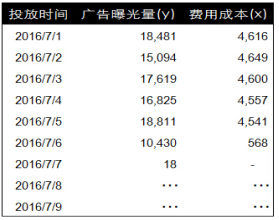第一种相关分析方法是将数据进行可视化处理，简单的说就是绘制图表。单纯从数据的角度很难发现其中的趋势和联系，而将数据点绘制成图表后趋势和联系就会变的清晰起来。

对于有明显时间维度的数据，我们选择使用折线图。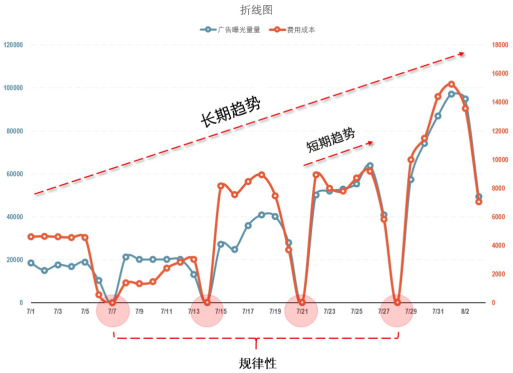为了更清晰的对比这两组数据的变化和趋势，我们使用双坐标轴折线图，其中主坐标轴用来绘制广告曝光量数据，次坐标轴用来绘制费用成本的数据。通过折线图可以发现，费用成本和广告曝光量两组数据的变化和趋势大致相同，从整体的大趋势来看，费用成本和广告曝光量两组数据都呈现增长趋势。从规律性来看费用成本和广告曝光量数据每次的最低点都出现在同一天。从细节来看，两组数据的短期趋势的变化也基本一致。

比折线图更直观的是散点图。散点图去除了时间维度的影响，只关注广告曝光量和费用成本这里两组数据间的关系。在绘制散点图之前，我们将费用成本标识为X，也就是自变量，将广告曝光量标识为y，也就是因变量。下面是一张根据每一天中广告曝光量和费用成本数据绘制的散点图，X轴是自变量费用成本数据，Y轴是因变量广告曝光量数据。从数据点的分布情况可以发现，自变量x和因变量y有着相同的变化趋势，当费用成本的增加后，广告曝光量也随之增加。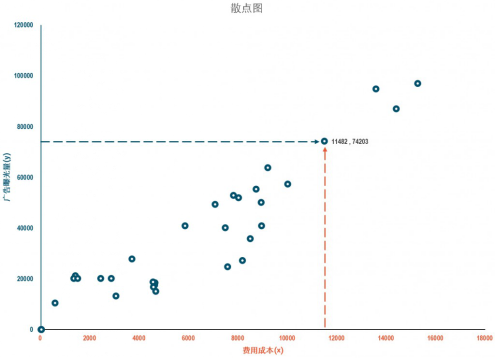折线图和散点图都清晰的表示了广告曝光量和费用成本两组数据间的相关关系，优点是对相关关系的展现清晰，缺点是无法对相关关系进行准确的度量，缺乏说服力。并且当数据超过两组时也无法完成各组数据间的相关分析

2，协方差及协方差矩阵

第二种相关分析方法是计算协方差。协方差用来衡量两个变量的总体误差，如果两个变量的变化趋势一致，协方差就是正值，说明两个变量正相关。如果两个变量的变化趋势相反，协方差就是负值，说明两个变量负相关。如果两个变量相互独立，那么协方差就是0，说明两个变量不相关。以下是协方差的计算公式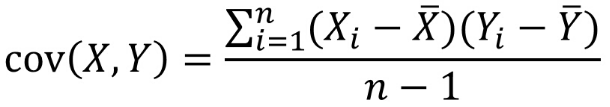下面是广告曝光量和费用成本间协方差的计算过程和结果，经过计算，我们得到了一个很大的正值，因此可以说明两组数据间是正相关的。广告曝光量随着费用成本的增长而增长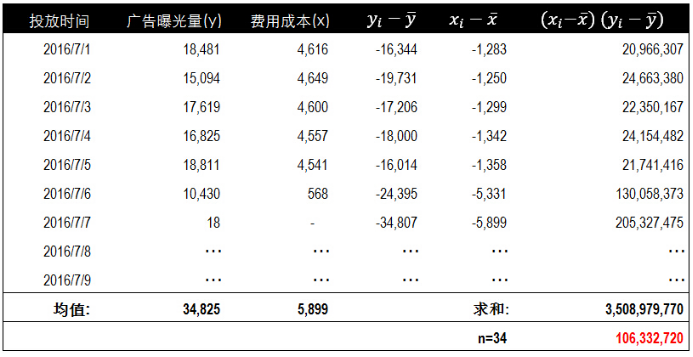协方差只能对两组数据进行相关性分析，当有两组以上数据时就需要使用协方差矩阵。下面是三组数据x，y，z，的协方差矩阵计算公式。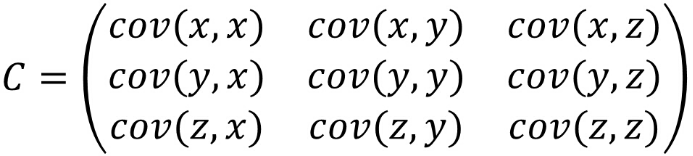协方差通过数字衡量变量间的相关性，正值表示正相关，负值表示负相关。但无法对相关的密切程度进行度量。当我们面对多个变量时，无法通过协方差来说明那两组数据的相关性最高。要衡量和对比相关性的密切程度，就需要使用下一个方法：相关系数

3，相关系数

第三个相关分析方法是相关系数。相关系数(Correlation coefficient)是反应变量之间关系密切程度的统计指标，相关系数的取值区间在1到-1之间。1表示两个变量完全线性相关，-1表示两个变量完全负相关，0表示两个变量不相关。数据越趋近于0表示相关关系越弱。以下是相关系数的计算公式。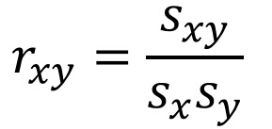其中rxy表示样本相关系数，Sxy表示样本协方差，Sx表示X的样本标准差，Sy表示y的样本标准差。下面分别是Sxy协方差和Sx和Sy标准差的计算公式。由于是样本协方差和样本标准差，因此分母使用的是n-1。

Sxy样本协方差计算公式：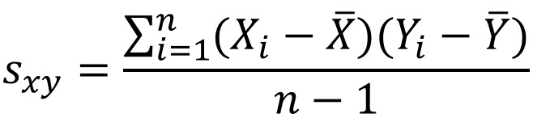Sx样本标准差计算公式：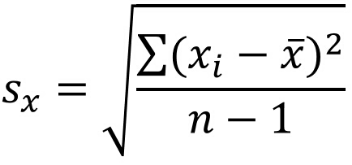Sy样本标准差计算公式：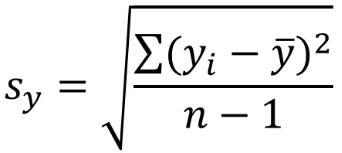下面是计算相关系数的过程，在表中我们分别计算了x，y变量的协方差以及各自的标准差，并求得相关系数值为0.93。0.93大于0说明两个变量间正相关，同时0.93非常接近于1，说明两个变量间高度相关。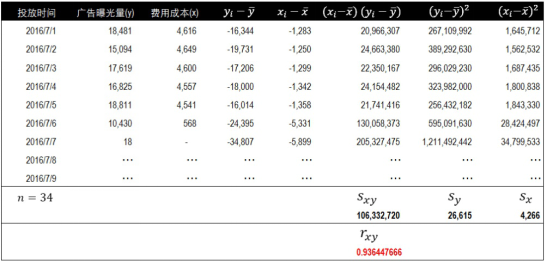在实际工作中，不需要上面这么复杂的计算过程，在Excel的数据分析模块中选择相关系数功能，设置好x，y变量后可以自动求得相关系数的值。在下面的结果中可以看到，广告曝光量和费用成本的相关系数与我们手动求的结果一致。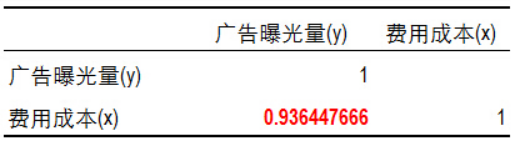相关系数的优点是可以通过数字对变量的关系进行度量，并且带有方向性，1表示正相关，-1表示负相关，可以对变量关系的强弱进行度量，越靠近0相关性越弱。缺点是无法利用这种关系对数据进行预测，简单的说就是没有对变量间的关系进行提炼和固化，形成模型。要利用变量间的关系进行预测，需要使用到下一种相关分析方法，回归分析

4，一元回归及多元回归

第四种相关分析方法是回归分析。回归分析（regression analysis)是确定两组或两组以上变量间关系的统计方法。回归分析按照变量的数量分为一元回归和多元回归。两个变量使用一元回归，两个以上变量使用多元回归。进行回归分析之前有两个准备工作，第一确定变量的数量。第二确定自变量和因变量。我们的数据中只包含广告曝光量和费用成本两个变量，因此使用一元回归。根据经验广告曝光量是随着费用成本的变化而改变的，因此将费用成本设置为自变量x，广告曝光量设置为因变量y。

以下是一元回归方程，其中y表示广告曝光量，x表示费用成本。b0为方程的截距，b1为斜率，同时也表示了两个变量间的关系。我们的目标就是b0和b1的值，知道了这两个值也就知道了变量间的关系。并且可以通过这个关系在已知成本费用的情况下预测广告曝光量。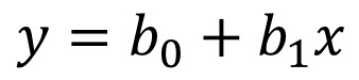这是b1的计算公式，我们通过已知的费用成本x和广告曝光量y来计算b1的值。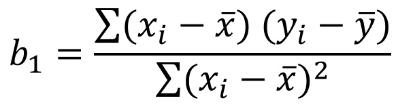以下是通过最小二乘法计算b1值的具体计算过程和结果，经计算，b1的值为5.84。同时我们也获得了自变量和因变量的均值。通过这三个值可以计算出b0的值。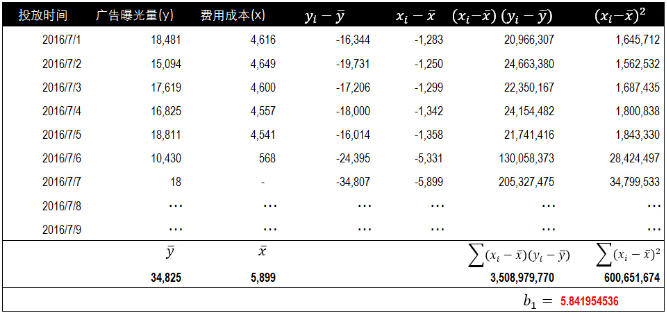以下是b0的计算公式，在已知b1和自变量与因变量均值的情况下，b0的值很容易计算。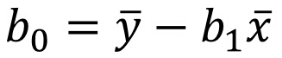将自变量和因变量的均值以及斜率b1代入到公式中，求出一元回归方程截距b0的值为374。这里b1我们保留两位小数，取值5.84。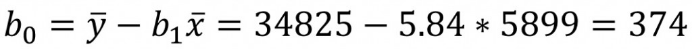在实际的工作中不需要进行如此繁琐的计算，Excel可以帮我们自动完成并给出结果。在Excel中使用数据分析中的回归功能，输入自变量和因变量的范围后可以自动获得b0（Intercept）的值362.15和b1的值5.84。这里的b0和之前手动计算获得的值有一些差异，因为前面用于计算的b1值只保留了两位小数。

这里还要单独说明下R Square的值0.87。这个值叫做判定系数，用来度量回归方程的拟合优度。这个值越大，说明回归方程越有意义，自变量对因变量的解释度越高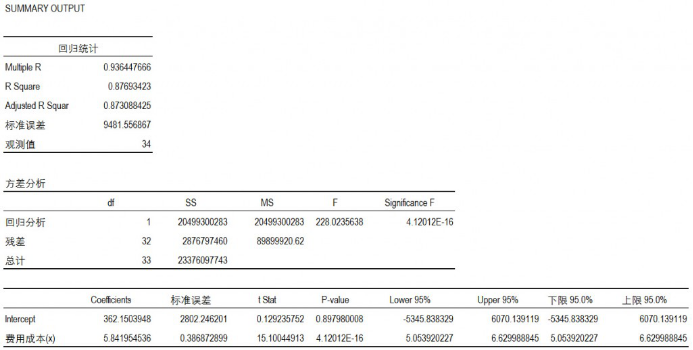将截距b0和斜率b1代入到一元回归方程中就获得了自变量与因变量的关系。费用成本每增加1元，广告曝光量会增加379.84次。通过这个关系我们可以根据成本预测广告曝光量数据。也可以根据转化所需的广告曝光量来反推投入的费用成本。获得这个方程还有一个更简单的方法，就是在Excel中对自变量和因变量生成散点图，然后选择添加趋势线，在添加趋势线的菜单中选中显示公式和显示R平方值即可。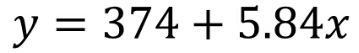以上介绍的是两个变量的一元回归方法，如果有两个以上的变量使用Excel中的回归分析，选中相应的自变量和因变量范围即可。下面是多元回归方程。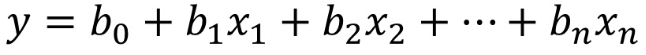5，信息熵及互信息

最后一种相关分析方法是信息熵与互信息。前面我们一直在围绕消费成本和广告曝光量两组数据展开分析。实际工作中影响最终效果的因素可能有很多，并且不一定都是数值形式。比如我们站在更高的维度来看之前的数据。广告曝光量只是一个过程指标，最终要分析和关注的是用户是否购买的状态。而影响这个结果的因素也不仅仅是消费成本或其他数值化指标。可能是一些特征值。例如用户所在的城市，用户的性别，年龄区间分布，以及是否第一次到访网站等等。这些都不能通过数字进行度量。

度量这些文本特征值之间相关关系的方法就是互信息。通过这种方法我们可以发现哪一类特征与最终的结果关系密切。下面是我们模拟的一些用户特征和数据。在这些数据中我们忽略之前的消费成本和广告曝光量数据，只关注特征与状态的关系。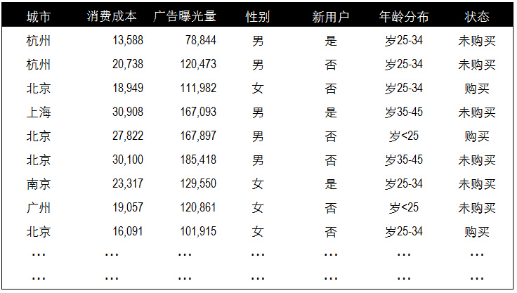回归方程可以对相关关系进行提炼，并生成模型用于预测，如何进行学生成绩情况与学生个人发展之间的关系分析，以回归分析为主，辅以图表分析，如果要对学生的个人情况进行更加深全面的预测，在情况比较复杂的情况下，需要用到信息熵与互信息


展开全文• 今天跟大家来分享的是另外一种比较常见的分析—相关性分析。相关分析是研究两个或两个以上处于同等地位的随机变量间的相关关系的统计分析方法。比如我们在研究人的身高体重之间关系的时候，我们就会用到相关性分析。...


昨天跟大家探讨了如何使用SPSS进行方差分析，知道了方差分析的原理以及最后的结果如何解读。今天跟大家来分享的是另外一种比较常见的分析—相关性分析。相关分析是研究两个或两个以上处于同等地位的随机变量间的相关关系的统计分析方法。比如我们在研究人的身高体重之间关系的时候，我们就会用到相关性分析。说到这里，大家是不是会想到一个比较熟悉的方法—回归分析。其实两种分析是不一样的，相关性分析偏向于发现自变量之间的相关性。而回归分析偏向于自变量的变化是如何导致因变量的变化。不过在实际分析操作中，回归分析和相关分析有着密切的关系。下面，我们就进入到今天的相关分析：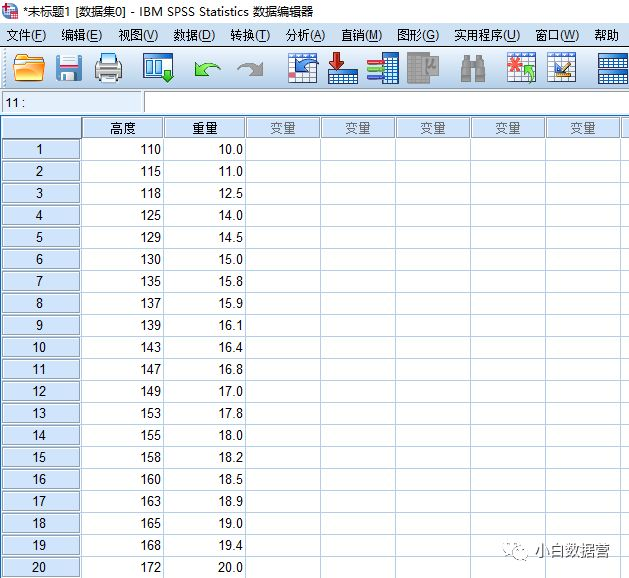上图就是本次分析所用到的数据。两列数据分别代表植物的生长高度以及与之对应的重量，我们需要通过相关分析来研究两者之间是否具有相关性，如果具有相关性又是怎样的一种相关关系。我们从分析选项栏中选择相关，然后选择双变量，我们就可以进入到相关性分析的界面了：我们将两个变量选中以后，下方需要选择Pearson相关系数(一般会是默认选项，主要通过Pearson相关系数来判断相关性)，其次我们在显著性检验中选择双侧检验，下面勾选标记显著性相关。在这次的分析中，小白还在右边的选项栏里的统计量选项中勾选了均值与标准差：其它选项我们均按系统默认的就行，不用进行更改，点击确定以后就会出现本次相关分析的结果：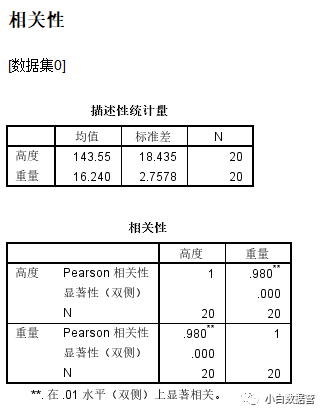从上图中我们可以看到，第一个统计量的表格就是系统针对本次数据进行的描述性统计。下方就是相关分析的结果，从结果中我们可以看到，植物生长高度和重量之间的Pearson相关系数为0.980，一般来说，Pearson相关系数的取值是-1到1，分别对应负相关以及正相关，当Pearson相关系数的绝对值越趋近于1的时候，相关性就越强。这里给大家列举一下Pearson相关系数的结果判定：1.当系数在0.8-1.0时，两者存在极强相关性；2.当系数在0.6-0.8时，两者存在强相关性；3.当系数在0.4-0.6时，两者中等程度相关；4.当系数在0.2-0.4时，两者存在弱相关性；5.当系数在0.0-0.2时，两者极弱相关或无相关性。从这个我们可以判定，植物的生长高度同它们重量之间存在极强的正相关性。同时我们在表中也可以看到，显著性P值为0.000<0.05，说明两者之间存在显著性差异，下方的N为样本量大小。到这里，我们的相关分析就完成了，其实这只是一个比较简单的例子，但是在实际工作中，基本上都是会有很多因素堆积在一起，我们无法直观的去了解哪些变量之间存在相关性，这个时候，我们就需要用相关分析来快速并且准确的找到我们所需要的变量。每天五分钟，轻松积累数据分析知识讨论QQ群：514581193数据干货，各种福利，不定期发放

展开全文• 两个变量与因变量相关性分析提问：用SPSS一个分析,有一个因变量和N个自变量,先做相关性发现有很多自变量与因变量有关,相关性也比较高.继续说,但是再做多重回归方程的时候只有3个因变量入选,其他都被排除了,那在写...
两个变量与因变量相关性分析提问：用SPSS一个分析,有一个因变量和N个自变量,先做相关性发现有很多自变量与因变量有关,相关性也比较高.继续说,但是再做多重回归方程的时候只有3个因变量入选,其他都被排除了,那在写文章的时候那些被排除了的有相关性的因变量该怎么处理呢?这说明这些变量之间存在自相关,模型选择的是代表程度更高且自变量相互之间相关性低的自变量来,以保证自变量变化时,只影响因变量,而不影响其它模型中的自变量.建议你对这些自变量做两两之间的相关性检验,以说明他们不适合同时存在于模型中.追问：这个是所谓的共线性的问题么？那我做自变量两两之间的相关性检验，什么样的结果才能显示他们不适合同时出现在模型中呢？追答：你进行自变量之间的相关性检验，结果就会出来他们之间的相关性很高。 至于具体到模型中，得看具体的情况了，我也没有经验值。但是建模的时候一定要选择合适的变量进入方式。最佳答案：1.多重共线性的概念：所谓多重共线性(Multicollinearity)是指线性回归模型中的解释变量之间由于存在精确相关关系或高度相关关系而使模型估计失真或难以估计准确。一般来说，由于经济数据的限制使得模型设计不当，导致设计矩阵中解释变量间存在普遍的相关关系。完全共线性的情况并不多见，一般出现的是在一定程度上的共线性，即近似共线性。2.多重共线性产生的原因 　　主要有3各方面： 　　(1)经济变量相关的共同趋势 　　(2)滞后变量的引入 　　(3)样本资料的限制 3多重共线性的解决方法多重共线性的处理方法一般有如下的几种1 增加样本容量,当线性重合是由于测量误差引起的以及他仅是偶然存在于原始样本,而不存在于总体时,通过增加样本容量可以减少或是避免线性重合,但是在现实的生活中,由于受到各种条件的限制增加样本容量有时又是不现实的2剔除一些不重要的解释变量,主要有向前法和后退法,逐步回归法.前进法的主要思想是变量由少到多的,每次增加一个,直至没有可引入的变量为止.具体做法是首先对一个因变量y和m个自变量分别建立回归方程,并分别计算这m个回归方程的F值,选其最大者,记为Fj,,给定显著性水平F,如果Fj>F,则变量引入该方程,再分别对(Xj,X1),(Xj,X2)…(Xj,Xm)做回归方程,并对他们进行F检验,选择最大的Fi值,如果Fi.>F,则该变量引入方程,重复上述步骤,直到没有变量引入为止.后退法,是先用m个因变量建立回归方程,然后在这m个变量中选择一个最不显著的变量将它从方程中剔除,对m个回归系数进行F检验,记所求得的最小的一个记为Fj,给定一个显著性的水平,如果Fj逐步回归法,前进法存在着这样的缺点当一个变量被引入方程时,这个变量就被保留在这个方程中了,当引入的变量导致其不显著时,它也不会被删除掉,后退法同样存在着这样的缺点,当一个变量被剔除时就永远的被排斥在方程以外了,而逐步回归法克除了两者的缺点.逐步回归的思想是有进有出.将变量一个一个的引入,每引入一个变量对后面的变量进行逐个检验,当变量由于后面变量的引入而不变的不显著时将其剔除,进行每一步都要进行显著性的检验,以保证每一个变量都是显著的.理论上上面的三种方法都是针对不相关的的数据而言的,在多重共线性很严重的情况下,结论 的可靠性受到影响,在一些经济模型中,要求一些很重要变量必须包含在里面,这时如果贸然的删除就不符合现实的经济意义.3.不相关的系数法.当变量之间存在着多重共线性最直接的表现就是各个解释变量之间的决定系数很大.考虑到两个变量之间的决定系数众所周知, 在多元线性回归模型中, 当各个解释变量( 如Xi 与Xj, i≠j) 之间存在着多重共线性时, 其最直接的表现就是各个解释变量之间的决定系数(ri2,j)很大.ri2,j 很大, 则意味着重要变量Xi( 在本文中, 为研究方便, 我们始终假定Xi 相对于Xj 而言, 是一重要变量, i≠j) 的变化能够说明Xj 的变化.如两者之间的r2,j=90%, 则我们以说, Xi 的变化说明了Xj 变化的90%,而剩余的( 1- ri2,j) 部分,则是由Xj 自身的变化说明的.由此决定, 在反映被解释变量(Y)与解释变量Xi,Xj 之间的关系时, 对于解释变量Xj 来说, 并不需要用全部的信息来解释被解释变量的问题, 而只需要用剩余的( 1- ri2,j) 部分的信息来解释就足够了,因为有ri2,j 部分的信息是与Xi 相重复的, 已由Xi 解释了.由此出发, 如果我们能够在保留重要变量(Xi) 全部信息的同时, 以重要变量(Xi) 为基础, 对其他的解释变量进行一定的线形变换, 使之转换为一个新变量, 如将Xj 转换为Xjj , 并且使得Xi 与新变量Xjj 之间的决定系数( ri2,jj) 降低到最小程度———如( 1- ri2,j) , 则就可以消除多重共线性.喜欢 (5)or分享 (0)
展开全文• 内容目录Pearson相关系数(积差相关系数)适用条件Spearman等级相关系数适用条件Kendall's Tau相关系数适用条件偏相关适用条件R语言实现Pearson、Spearman、Kendall示例偏相关相关性显著性检验相关性可视化相关性分析......